Learn All Concepts of Chapter 1 Class 9 - FREE. Check - Chapter 1 Class 9 Maths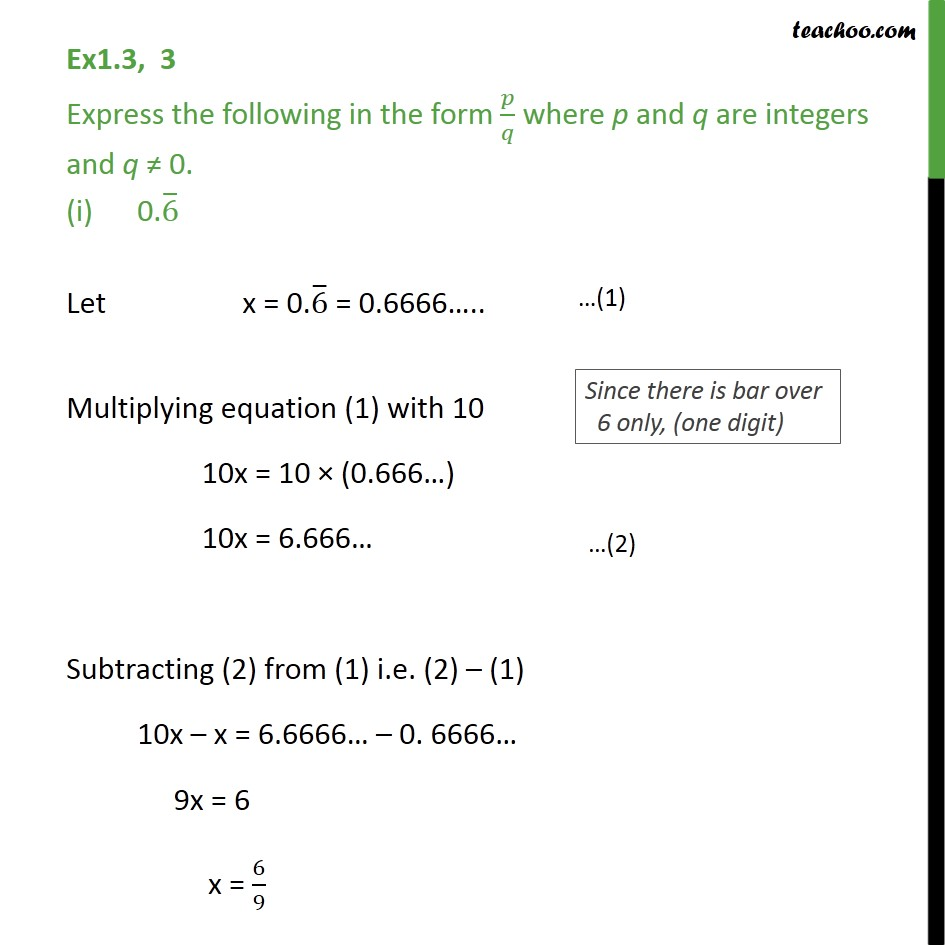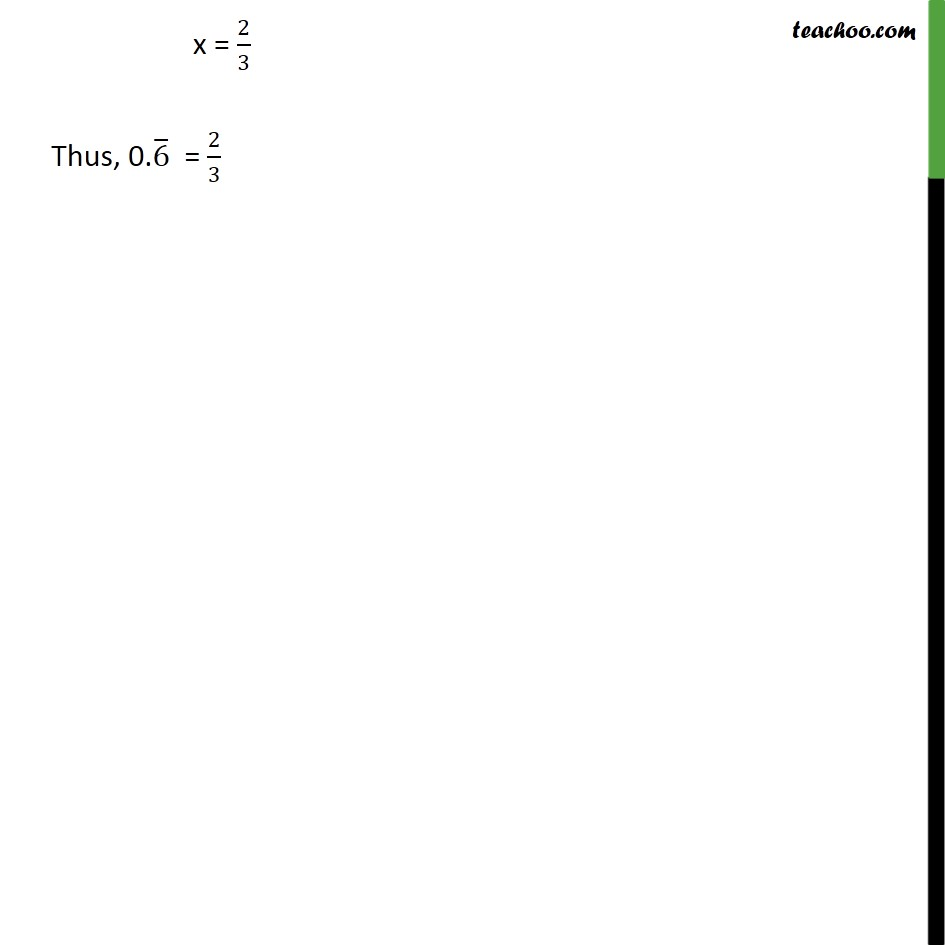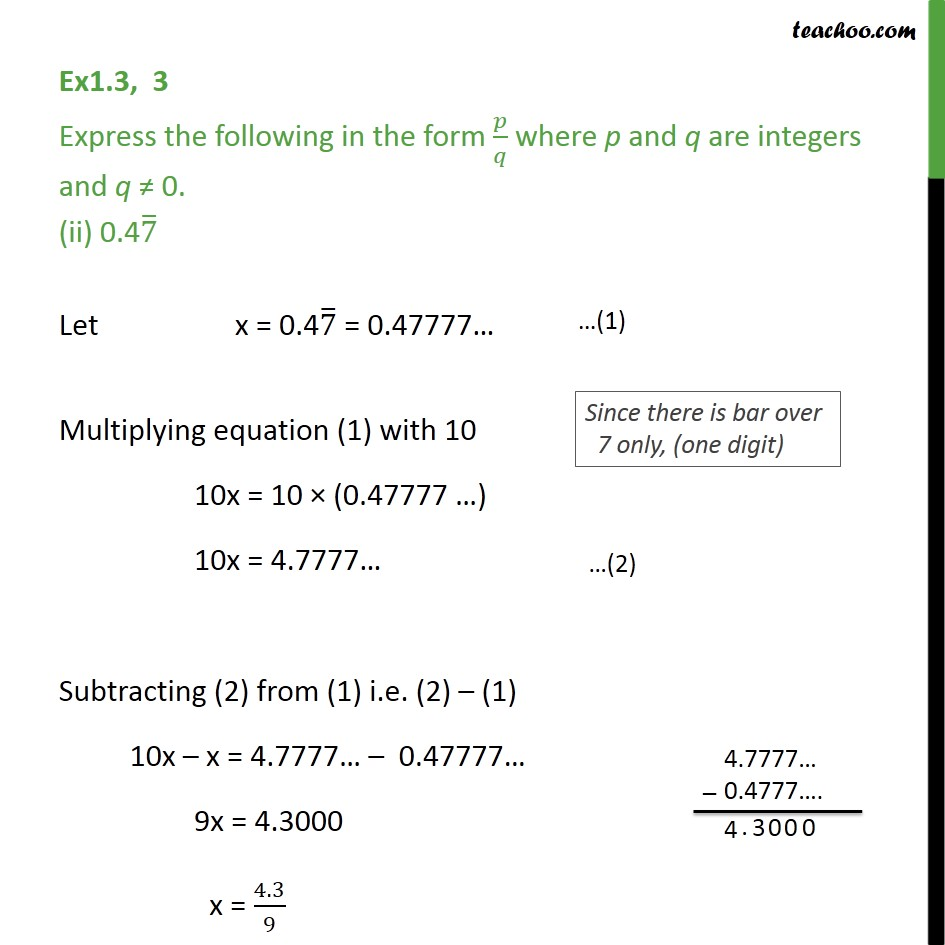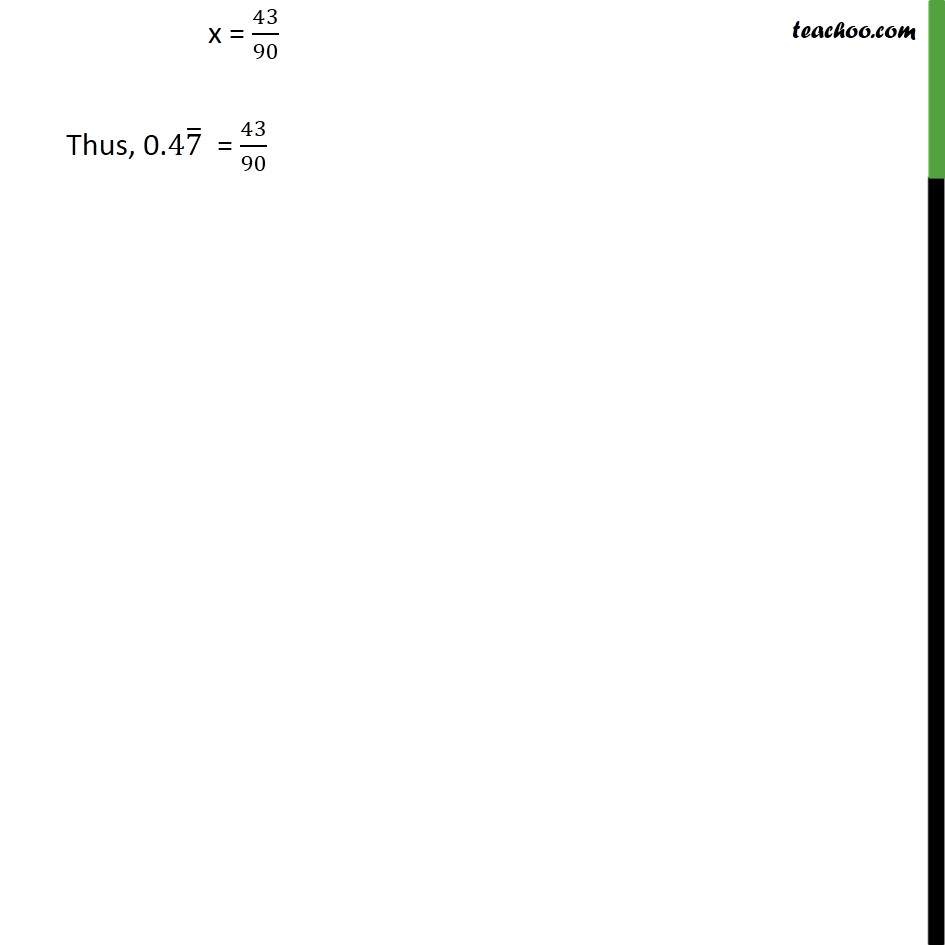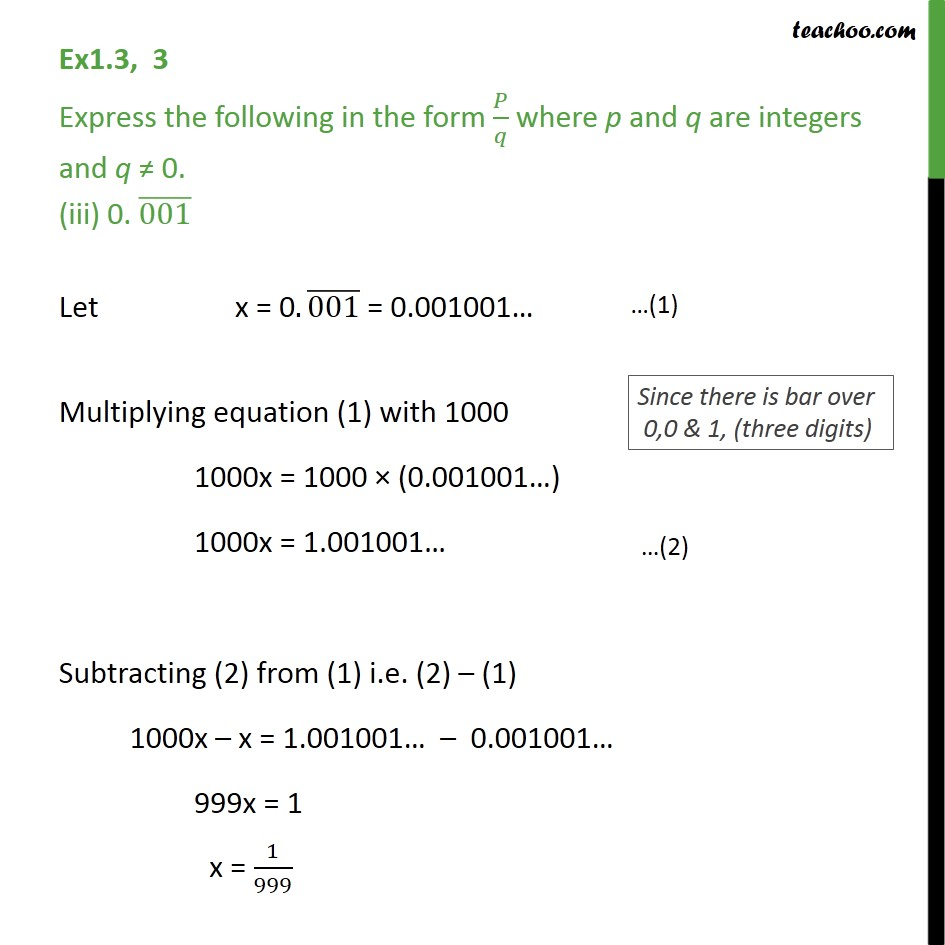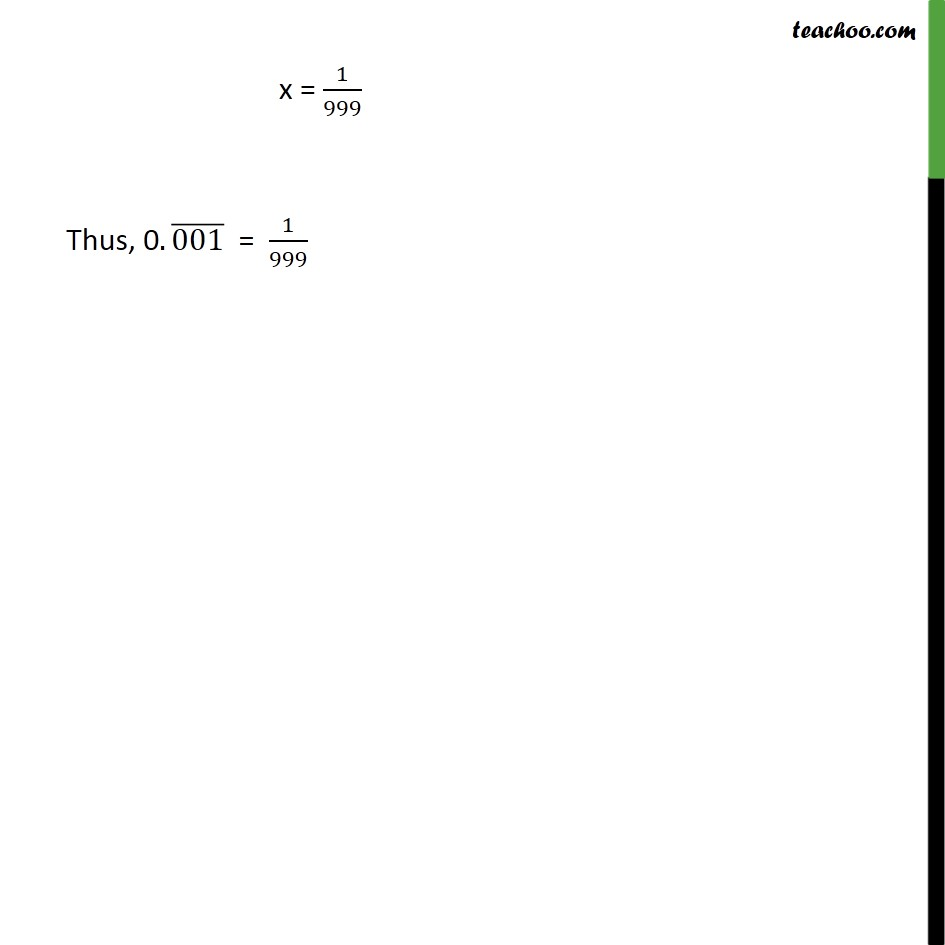1. Chapter 1 Class 9 Number Systems
2. Serial order wise
3. Ex 1.3

Transcript

Ex1.3, 3 Express the following in the form 𝑝/𝑞 where p and q are integers and q ≠ 0. 0.6 ̅ Let x = 0.6 ̅ = 0.6666….. Multiplying equation (1) with 10 10x = 10 × (0.666…) 10x = 6.666… Subtracting (2) from (1) i.e. (2) – (1) 10x – x = 6.6666… – 0. 6666… 9x = 6 x = 6/9 x = 2/3 Thus, 0.6 ̅ = 2/3 Ex1.3, 3 Express the following in the form 𝑝/𝑞 where p and q are integers and q ≠ 0. (ii) 0.47 ̅ Let x = 0.47 ̅ = 0.47777… Multiplying equation (1) with 10 10x = 10 × (0.47777 …) 10x = 4.7777… Subtracting (2) from (1) i.e. (2) – (1) 10x – x = 4.7777… – 0.47777… 9x = 4.3000 x = 4.3/9 x = 43/90 Thus, 0.47 ̅ = 43/90 Ex1.3, 3 Express the following in the form 𝑃/𝑞 where p and q are integers and q ≠ 0. (iii) 0. (001) ̅ Let x = 0.(001) ̅ = 0.001001… Multiplying equation (1) with 1000 1000x = 1000 × (0.001001…) 1000x = 1.001001… Subtracting (2) from (1) i.e. (2) – (1) 1000x – x = 1.001001… – 0.001001… 999x = 1 x = 1/999 x = 1/999 Thus, 0.(001) ̅ = 1/999

Ex 1.3